# Construction of a Rounded Skew-Superelevation

Basil Psarianos* and Konstantinos Apostoleris

National Technical University of Athens, Greece

Received Date: May 06, 2019;  Published Date: May 22, 2019

#### Introduction

Crash analysis of vehicle loss of control has shown that entering a curve constitutes a road section with high potential for developing hydroplaning [1-4]. This is due to the fact that at these sections, cross-slope rates reach small values (less than 0.5%) much less than those required for adequate drainage. If the existing longitudinal slope at this section is not adequate to provide a compound slope enough for a sufficient water drainage, the developed water film depth may become critical for hydroplaning. Ressel & Herrmann  have found that the crash risk at those locations is five times higher when the pavement becomes wet vs dry pavement conditions. Hydroplaning is a very complex phenomenon [3,4] and from the nine internationally available techniques to counteract the phenomenon, skew superelevation as illustrated in Figure 1 is one of the most effective [3,5,6]. Pavement skew superelevation is a technique that goes back into the ‘70s  and retained also in newer editions [7-9] aiming at diminishing any pavement section with a superelevation rate less than the minimum absolute value of 2.5% for European Guides or 2.0% for the AASHTO Green Book. The length of the skew superelevation transition from -2.5 (-2.0) % to +2.5 (2.0) % (see also Figure 1) is calculated according to the following Equation 1 [7,8] (Figure 1):Where: Lv [m] Length of skew superelevation runoff

B [m] Directional pavement width

Ve [km/h] Design speedIn the past some safety and riding comfort concerns have been raised as of its performance [3,7,8,11]. Lippold et al.  are probably the first ones who have addressed these concerns recently extensively followed by Apostoleris et al. [7,8,11]. With respect to the issue of road safety both studies showed that for travel speeds equal to the design speed no safety deficiencies of the skew superelevation exist. In addition, the newer investigation  based both on a simulation program as well as actual measurements on newly constructed skew super elevated sections on the freeway connecting cities Korinth and Patras in Greece provided with a roundup of the resulting edge of the skew superelevation using a vertical crest radius of H=100m, revealed a significant reduction both in the lateral and vertical accelerations being exerted on the driver when riding over it. The construction details of a skew superelevation rounded design is shown in Figures 2 & 3. The applied practice presents some difficulties during construction, especially in critical areas shown in Figure 2, thus making compaction of asphalt layers more complex in addition to the fact that at both terminals of the skew superelevation area the superelevation rates drop below 2.5% due to shortening of the tangent length of the crest vertical curve of the roundup in the critical area (Figures 2 & 3).#### Equations Regarding Skew Superelevation Rounded Design

The typical construction already applied for the rounded section of the skew superelevation has a width of 5.0m, by applying a radius of H = 100m. The results obtained after pertinent research through simulations , leads to the conclusion that rounding application with a shorter total width (vertical curve length) of 2.5m could be applied since it is not affecting the comfort and safety of riding vehicles over the rounded skew superelevation (Figure 4).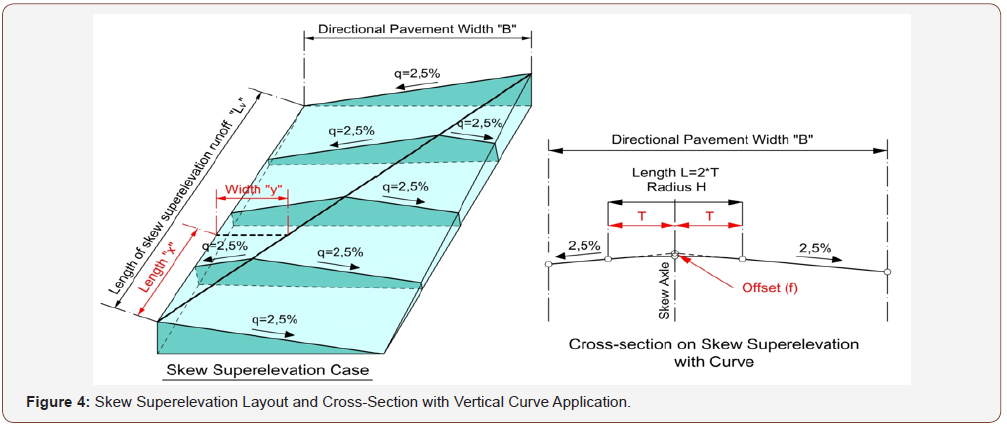The position of the skew superelevation edge, is calculated according to the following mathematical equation: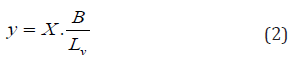Where: x [m] Longitudinal distance from the beginning of skew superelevation runoff

y [m] Lateral distance from the beginning of skew superelevation runoff

L [m] Length of skew superelevation runoff

B [m] Directional pavement width

The calculations of the Semi-Length T and the offset f parameters is done by using the mathematical equations applicable in longitudinal profile curves, by taking into account each one of side slopes equal to 2.5% (or 2.0% for AASHTO regulations). These equations are: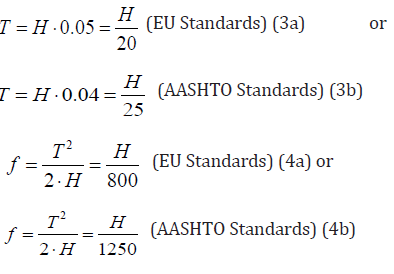Where: T [m] Curved Semi-Length

f [m] The offset in the middle of the curve

H [m] The radius applied on the curveRespectively, the offset z at any point of the curve can be calculated as follows: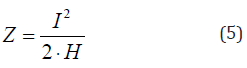Where: l [m] Distance from the beginning of the curve

z [m] Elevation difference from curve to tangent

Dz [m] Elevation difference from the beginning of the curve

H [m] The radius applied on the curve

#### Rounded Skew Superelevation Construction in Practice

The construction of the rounded skew superelevation is particularly difficult. In practice the rounded area is constructed in 4-6 strips of variable cross-slope, thus approaching the theoretical curve through a sequence of 4-6 chords. There were two cases examined.

##### Case I: Large curve applied / 4.00m~5.00m curve length

For large curve applied, with curve length between 4.00m~5.00m, the rounded area is suggested to be replaced by 6 strips with variable cross-slope as presented in Figures 6a (EU Regulations) & 6b (AASHTO Regulations) (Figures 6a,6b).##### Case II: Small curve applied / 2.50m~4.00m curve length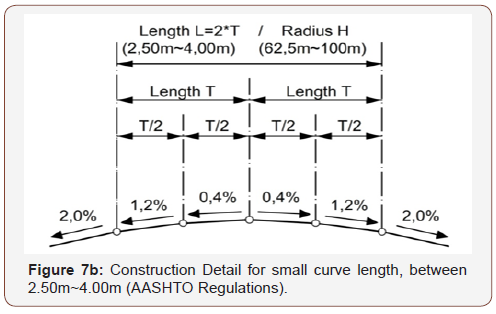For small curve applied, with curve length between 2.50m~4.00m, the rounded area is suggested to be replaced by 4 strips with variable cross-slope as presented in Figures 7a (EU Regulations) & 7b (AASHTO Regulations) (Figures 7a,7b).

#### Conclusion

The improvement that can be achieved regarding hydroplaning phenomenon has been demonstrated by the implementation of the rounded skew superelevation runoff at several sections of constructed motorways in Greece and EU, as well as from the research that has been carried out so far. The overall conclusion is that the rounded skew superelevation runoff could present a permanent solution to significantly alleviate the hydroplaning phenomenon while the road still remains safe and comfortable for vehicles and drivers. It is therefore recommended as a countermeasure to effectively and permanently resolve the hydroplaning problem for the desired design speed of the road.

None.

#### Conflict of Interest

No conflict of interest.

Article Details
Citation
Keywords
Scroll to
Scroll to Top